# Natural logarithm of absolute value of sine function

This article is about a particular function from a subset of the real numbers to the real numbers. Information about the function, including its domain, range, and key data relating to graphing, differentiation, and integration, is presented in the article.
View a complete list of particular functions on this wiki

## Definition

This function is defined as the composite of the natural logarithm and the absolute value of sine function, which in turn is the composite of the absolute value function and the sine function.

## Key data

Item Value
default domain All real numbers except the integer multiples of$\pi$, where it approaches$-\infty$ from both sides
range All nonpositive real numbers, i.e., the set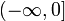$(-\infty,0]$.
absolute maximum value: 0
period$\pi$
important symmetries even function
mirror symmetry about any line of the form$x = n\pi/2, n \in \mathbb{Z}$
period of$\pi$
local maximum values and points of attainment local maximum values of 0 attained at all odd multiples of$\pi/2$
vertical asymptotes vertical asymptotes with both one-sided limits of$-\infty$ at all integer multiples of$\pi$
points of inflection (both coordinates) there are no points of inflection
first derivative cotangent function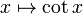$x \mapsto \cot x$
second derivative negative of cosecant-squared function, i.e.,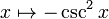$x \mapsto -\csc^2x$
antiderivative not among the elementary functions. Involves a polylogarithm
intervals of increase and decrease increasing on intervals of the form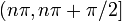$(n\pi,n\pi + \pi/2]$ where$n \in \mathbb{Z}$
decreasing on intervals of the form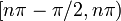$[n\pi - \pi/2,n\pi)$ where$n \in \mathbb{Z}$
intervals of concave up and concave down concave down on each of the intervals$(n\pi,n\pi + \pi)$ on which it is defined